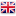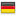# Sinergit Ltd.You are here:
25 May 2019

## Choice bumpers for tower crane out of service in headwinds

Value of the maximum reaction F on the two feedback at the limit of stabilityP = total weight of the crane strength (daN)

W = wind force (daN)

F = maximum force applied on the two feedback at the limit of stability (daN)

a = distance from the axis of tipping crane center of gravity (m)

c = distance from the axis of tilting force of the wind (m)

d = the center of gravity of the horizontal forces of inertia (m)

= center distance and strength of cranes inertia axis tilt (m)

Ms (bar) = P * a = stabilizing moment cranes

Mr (bar) = W * c = overturning moment cranes

F (daN) = (Ms - Mr) / e

NB. Each buffer will not have a maximum reaction above F / 2